# Financial market & institution CH3 .pptx

27 Feb 2023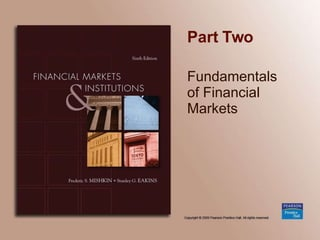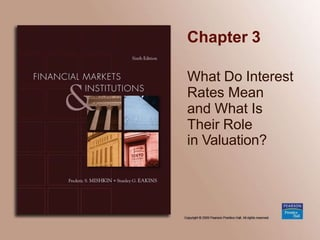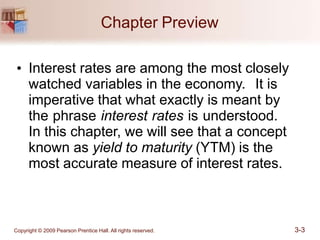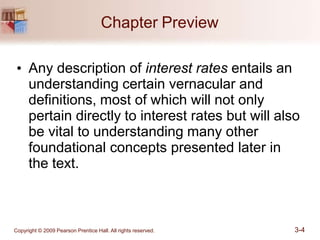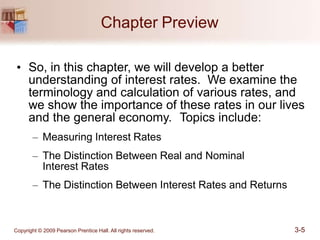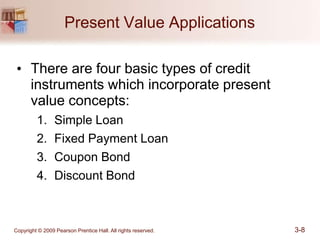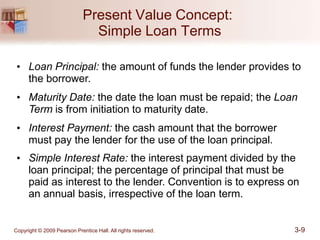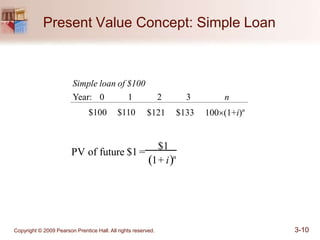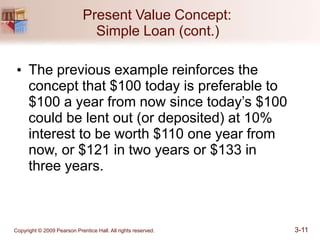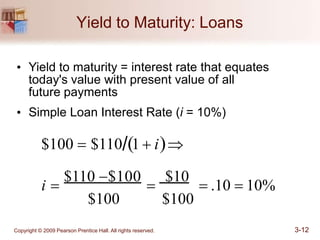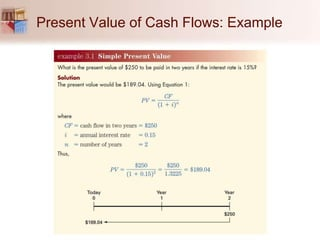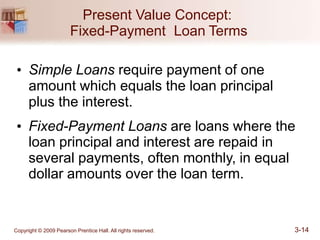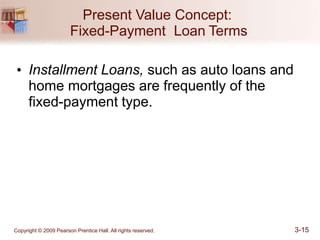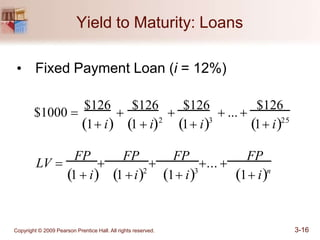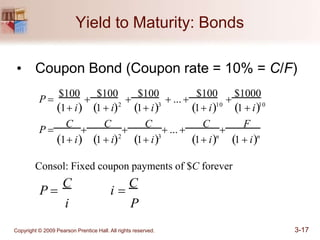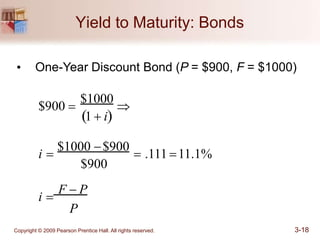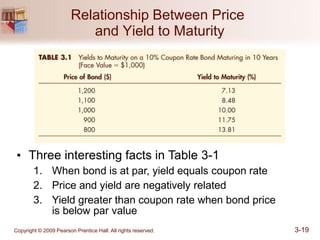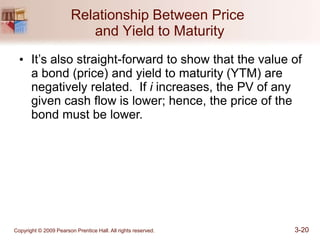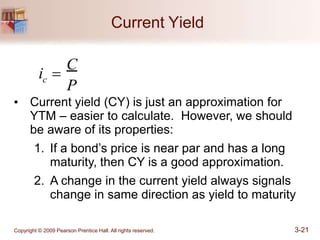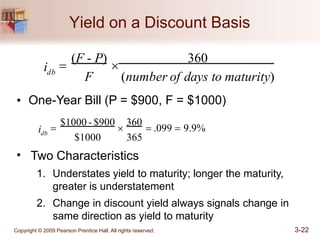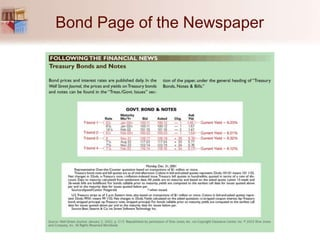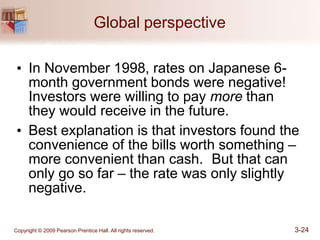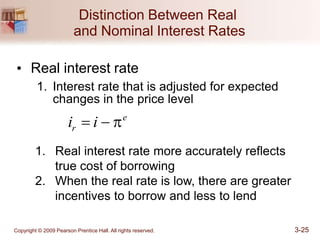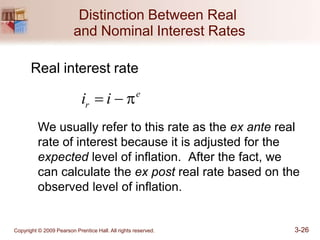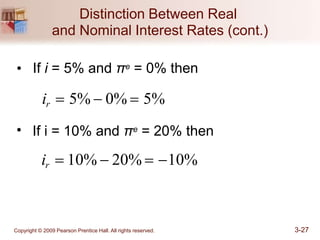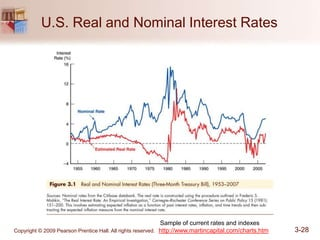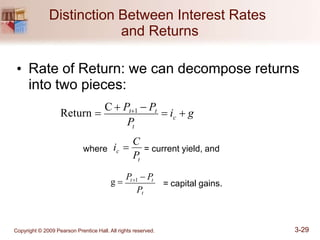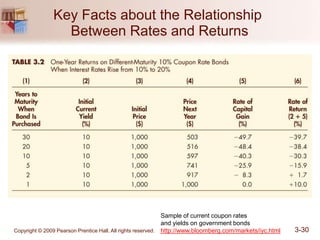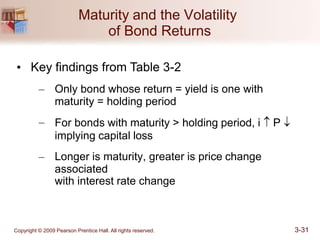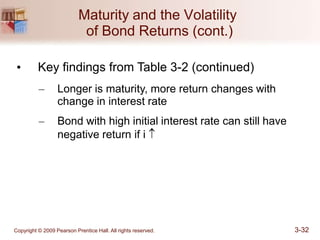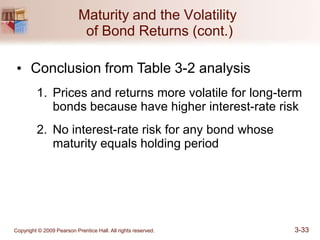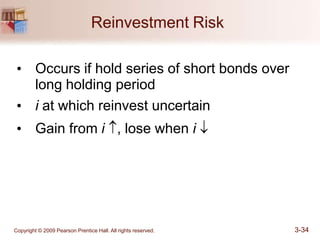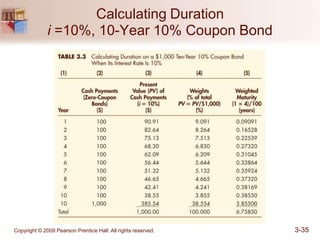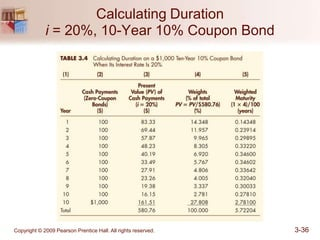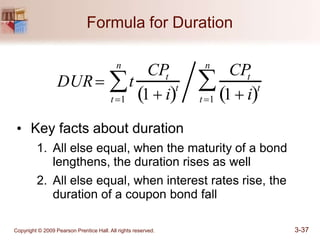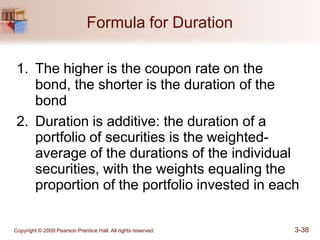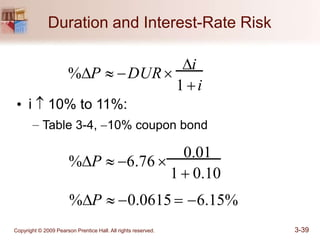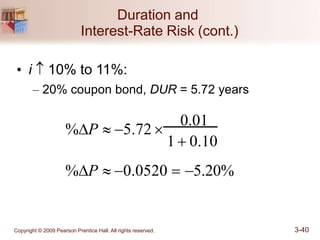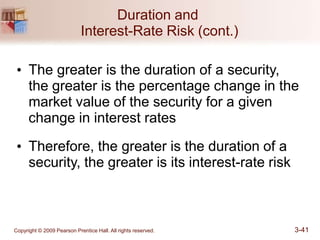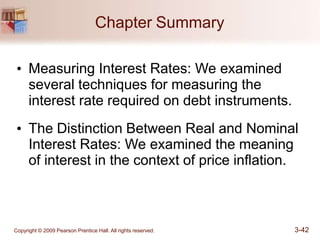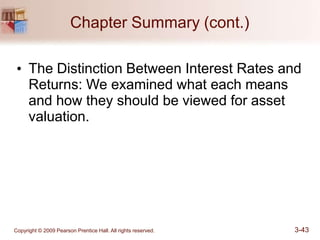1 sur 43

### Financial market & institution CH3 .pptx

• 2. Chapter 3 What Do Interest Rates Mean and What Is Their Role in Valuation?
• 3. Chapter Preview 3-3 Copyright © 2009 Pearson Prentice Hall. All rights reserved. • Interest rates are among the most closely watched variables in the economy. It is imperative that what exactly is meant by the phrase interest rates is understood. In this chapter, we will see that a concept known as yield to maturity (YTM) is the most accurate measure of interest rates.
• 4. Chapter Preview 3-4 Copyright © 2009 Pearson Prentice Hall. All rights reserved. • Any description of interest rates entails an understanding certain vernacular and definitions, most of which will not only pertain directly to interest rates but will also be vital to understanding many other foundational concepts presented later in the text.
• 5. Chapter Preview 3-5 Copyright © 2009 Pearson Prentice Hall. All rights reserved. • So, in this chapter, we will develop a better understanding of interest rates. We examine the terminology and calculation of various rates, and we show the importance of these rates in our lives and the general economy. Topics include: – Measuring Interest Rates – The Distinction Between Real and Nominal Interest Rates – The Distinction Between Interest Rates and Returns
• 6. Present Value Introduction 3-6 Copyright © 2009 Pearson Prentice Hall. All rights reserved. • Different debt instruments have very different streams of cash payments to the holder (known as cash flows), with very different timing. • All else being equal, debt instruments are evaluated against one another based on the amount of each cash flow and the timing of each cash flow. • This evaluation, where the analysis of the amount and timing of a debt instrument’s cash flows lead to its yield to maturity or interest rate, is called present value analysis.
• 7. Present Value 3-7 Copyright © 2009 Pearson Prentice Hall. All rights reserved. • The concept of present value (or present discounted value) is based on the commonsense notion that a dollar of cash flow paid to you one year from now is less valuable to you than a dollar paid to you today. This notion is true because you could invest the dollar in a savings account that earns interest and have more than a dollar in one year. • The term present value (PV) can be extended to mean the PV of a single cash flow or the sum of a sequence or group of cash flows.
• 8. Present Value Applications 3-8 Copyright © 2009 Pearson Prentice Hall. All rights reserved. • There are four basic types of credit instruments which incorporate present value concepts: 1. Simple Loan 2. Fixed Payment Loan 3. Coupon Bond 4. Discount Bond
• 9. Present Value Concept: Simple Loan Terms 3-9 Copyright © 2009 Pearson Prentice Hall. All rights reserved. • Loan Principal: the amount of funds the lender provides to the borrower. • Maturity Date: the date the loan must be repaid; the Loan Term is from initiation to maturity date. • Interest Payment: the cash amount that the borrower must pay the lender for the use of the loan principal. • Simple Interest Rate: the interest payment divided by the loan principal; the percentage of principal that must be paid as interest to the lender. Convention is to express on an annual basis, irrespective of the loan term.
• 10. Simple loan of \$100 2 3 n Year: 0 1 \$100 \$110 \$121 \$133 100(1+i)n PV of future \$1 = \$1 3-10 Copyright © 2009 Pearson Prentice Hall. All rights reserved. 1+ in Present Value Concept: Simple Loan
• 11. Present Value Concept: Simple Loan (cont.) • The previous example reinforces the concept that \$100 today is preferable to \$100 a year from now since today’s \$100 could be lent out (or deposited) at 10% interest to be worth \$110 one year from now, or \$121 in two years or \$133 in three years. 3-11 Copyright © 2009 Pearson Prentice Hall. All rights reserved.
• 12. Yield to Maturity: Loans • Yield to maturity = interest rate that equates today's value with present value of all future payments • Simple Loan Interest Rate (i = 10%) \$100  \$110 1  i i  \$110 \$100  \$10  .10  10% \$100 \$100 3-12 Copyright © 2009 Pearson Prentice Hall. All rights reserved.
• 13. Present Value of Cash Flows: Example
• 14. Present Value Concept: Fixed-Payment Loan Terms • Simple Loans require payment of one amount which equals the loan principal plus the interest. • Fixed-Payment Loans are loans where the loan principal and interest are repaid in several payments, often monthly, in equal dollar amounts over the loan term. 3-14 Copyright © 2009 Pearson Prentice Hall. All rights reserved.
• 15. Present Value Concept: Fixed-Payment Loan Terms • Installment Loans, such as auto loans and home mortgages are frequently of the fixed-payment type. 3-15 Copyright © 2009 Pearson Prentice Hall. All rights reserved.
• 16. Yield to Maturity: Loans 3-16 Copyright © 2009 Pearson Prentice Hall. All rights reserved. • Fixed Payment Loan (i = 12%) \$1000  \$126  \$126  \$126  ...  \$126 1 i 1  i2 1 i3 1 i25 LV  FP  FP  FP ...  FP 1  i 1 i2 1 i3 1 in
• 17. Yield to Maturity: Bonds 3-17 Copyright © 2009 Pearson Prentice Hall. All rights reserved. • Coupon Bond (Coupon rate = 10% = C/F) P  \$100  \$100  \$100  ...  \$100  \$1000 1 i 1  i2 1 i3 1 i10 1  i10 P  C  C  C  ...  C  F 1 i 1  i2 1 i3 1 in 1  in Consol: Fixed coupon payments of \$C forever P  C i  C i P
• 18. Yield to Maturity: Bonds 3-18 Copyright © 2009 Pearson Prentice Hall. All rights reserved. • One-Year Discount Bond (P = \$900, F = \$1000) 1  i \$900  \$1000  \$900 i  \$1000 \$900  .111 11.1% i  F  P P
• 19. Relationship Between Price and Yield to Maturity 3-19 Copyright © 2009 Pearson Prentice Hall. All rights reserved. • Three interesting facts in Table 3-1 1. When bond is at par, yield equals coupon rate 2. Price and yield are negatively related 3. Yield greater than coupon rate when bond price is below par value
• 20. Relationship Between Price and Yield to Maturity 3-20 Copyright © 2009 Pearson Prentice Hall. All rights reserved. • It’s also straight-forward to show that the value of a bond (price) and yield to maturity (YTM) are negatively related. If i increases, the PV of any given cash flow is lower; hence, the price of the bond must be lower.
• 21. Current Yield 3-21 Copyright © 2009 Pearson Prentice Hall. All rights reserved. • Current yield (CY) is just an approximation for YTM – easier to calculate. However, we should be aware of its properties: 1. If a bond’s price is near par and has a long maturity, then CY is a good approximation. 2. A change in the current yield always signals change in same direction as yield to maturity c i  C P
• 22. i 3-22 Copyright © 2009 Pearson Prentice Hall. All rights reserved. db  (F - P)  360 F (number of days to maturity) idb  \$1000 - \$900  360  .099  9.9% \$1000 365 • Two Characteristics 1. Understates yield to maturity; longer the maturity, greater is understatement 2. Change in discount yield always signals change in same direction as yield to maturity Yield on a Discount Basis • One-Year Bill (P = \$900, F = \$1000)
• 23. Bond Page of the Newspaper
• 24. Global perspective 3-24 Copyright © 2009 Pearson Prentice Hall. All rights reserved. • In November 1998, rates on Japanese 6- month government bonds were negative! Investors were willing to pay more than they would receive in the future. • Best explanation is that investors found the convenience of the bills worth something – more convenient than cash. But that can only go so far – the rate was only slightly negative.
• 25. Distinction Between Real and Nominal Interest Rates 3-25 Copyright © 2009 Pearson Prentice Hall. All rights reserved. • Real interest rate 1. Interest rate that is adjusted for expected changes in the price level r i  i  e 1. Real interest rate more accurately reflects true cost of borrowing 2. When the real rate is low, there are greater incentives to borrow and less to lend
• 26. Distinction Between Real and Nominal Interest Rates 3-26 Copyright © 2009 Pearson Prentice Hall. All rights reserved. Real interest rate r i  i  e We usually refer to this rate as the ex ante real rate of interest because it is adjusted for the expected level of inflation. After the fact, we can calculate the ex post real rate based on the observed level of inflation.
• 27. Distinction Between Real and Nominal Interest Rates (cont.) 3-27 Copyright © 2009 Pearson Prentice Hall. All rights reserved. • • If i = 5% and πe = 0% then ir  5%  0%  5% If i = 10% and πe = 20% then ir  10%  20%  10%
• 29. Copyright © 2009 Pearson Prentice Hall. All rights reserved. 3-29 Distinction Between Interest Rates and Returns • Rate of Return: we can decompose returns into two pieces: P c t Return  C  Pt1  Pt  i  g t c P where i  C = current yield, and Pt g  Pt1  Pt = capital gains.